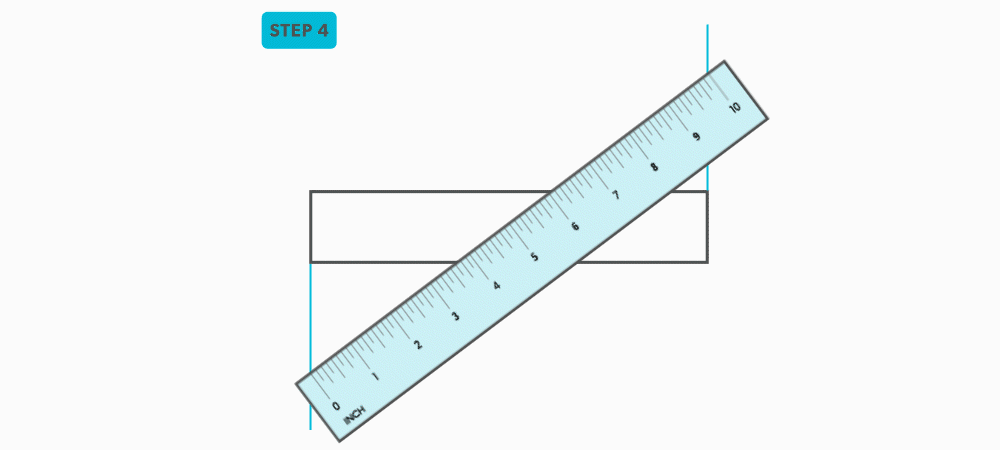Geometric Construction

# 10 Divide a Line Using a Ruler on an Angle

1. Draw a rectangle.2. Determine a larger length that divides easily into how many equal spaces you want. (For example, if four equal spaces are required, 10 inches, 12 inches, 16 inches, etc. are good choices.)3. Angle the ruler so that zero and the number chosen, in this case 10 inches, are on the , outside edges of the rectangle. (You may need to extend the vertical lines of the rectangle.)4. Mark the divisions along the ruler and square them vertically. If four divisions are required and 10 inches is chosen, then mark every 2.5 inches on the ruler.This is one of the most common techniques we use. Know it well!!!